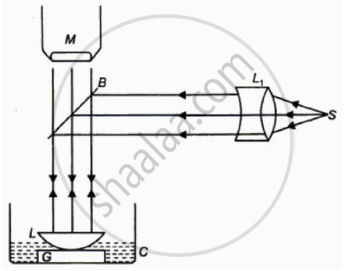# With Newton’s ring experiment explain how to determine the refractive index of liquid. - Applied Physics 2

Short Note

With Newton’s ring experiment explain how to determine the refractive index of liquid.

#### Solution

1.The experiment is performed when there is an air film between the plano-convex lens and the optically plane glass plate.2.The diametre of the mth and the (m+p)th dark rings are determined with the help of a travelling microscope.

For air :

D^(m+p^2) = 4(m+p) λR

Dm^2 = 4mλR

D(m+p^2)  - D_m^2= 4pλR

3.As shown in figure arrange the lens with glass plate. Pour one or two drops of liquid whose refractive index is to be determined without disturbing the arrangement. Now the air film between the lens and glass plate is replaced by the liquid. The diameters of m+pth and mth rings are determined.

For liquids,

2μtcos r = mλ,for dark rings

For normal incidence cosr =1,so

2μt = mλ

t = (r^2)/(2R)

r = D/2

Rearranging the above equation ,we get

D_m^2 =( 4mλR) /μ

We have D_(m+p)^2 - D_m^2 = 4pλR

For liquids,

D(m+p)^2 - D_m^2 = (4pλR)/μ

From these two euations the refractive index of the given liquids is given by

μ = (D_(m+p)^2−D_m^2 )/(D_(m+p)^('2) −D_m^('2)

4.In this way,refractive index of liquid is found using Newton’s rings.

Concept: Newton’S Rings
Is there an error in this question or solution?